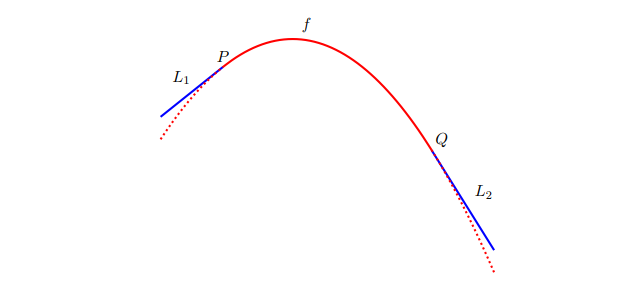# Sec.3.1 - Building a better roller coaster

Applied Project in Sec.3.1, Calculus by Stewart Chinese version: 建造較佳的雲霄飛車

Suppose you are asked to design the first ascent and drop for a new roller coaster. By studying the photographs of your favorite coasters, you decide to make the slope of the ascent $0.8$ and the slope of the drops $-1.6$. You decide to connect these two straight stretches $y=L_1(x)$ and $y=L_2(x)$ with part of a parabola $y=f(x) = ax^2+bx+c$, where $x$ and $f(x)$ are measured in meters. For the track to be smooth there can’t be abrupt changes in direction, so you want the linear segments $L_1$ and $L_2$ to be tangent to the parabola at the points $P$ and $Q$. To simplify the equations, you decide to place the origin at $P$.Question 1:

(a) Suppose the horizontal distance between $P$ and $Q$ is 30 m. Write equations in $a$, $b$, and $c$ that will ensure that the track is smooth at the transition points.

(b) Solve the equations in part(a) for $a$, $b$, and $c$ to find a formula for $f(x)$.

(c) Plot $L_1$, $f$, and $L_2$ to verify graphically that the transitions are smooth.

(d) Find the difference in elevation between $P$ and $Q$.

Below is the graph of these formula:

As you can see, the transition among $L_1$, $f$, and $L_2$ is smooth.

Question 2:

The solution in Problem 1 might look smooth, but it might not feel smooth because the piecewise defined function (consisting of $L_1(x)$ for $x<0$, $f(x)$ for $0\le x\le 30$, and $L_2(x)$ for $x>30$) doesn’t have a continuous second derivative. So you decide to improve the design by using a quadratic function $q(x)=ax^2 + bx + c$ only on the interval $3\le x\le 27$ and connecting it to the linear functions by means of two cubic functions: $$g(x) = kx^3+lx^2+mx+n, \quad 0\le x<3,$$ $$h(x) = px^3 + qx^2 + rx+s, \quad 27<x\leq 30.$$

(a) Write a system of equations in $11$ unknowns that ensure that the functions and their first two derivatives agree at the transition points.

(b) Solve the equations in part (a) with a computer algebra system to find formulas for $q(x)$, $g(x)$, and $h(x)$.

(c) Plot $L_1$, $g$, $q$, $h$, and $L_2$, and compare with the plot in Problem1(c).

The red one is the graph of $g(x)$, the blue one is the graph of $q(x)$, and the green one is the graph of $h(x)$.